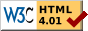#Boost C++ Libraries

...one of the most highly regarded and expertly designed C++ library projects in the world.

This is the documentation for an old version of boost. Click here for the latest Boost documentation.

# Disjoint Sets

```disjoint_sets<Rank, Parent, FindCompress>
```

This is class that provides disjoint sets operations with union by rank and path compression. A disjoint-sets data structure maintains a collection S = {S1, S2, ..., Sk} of disjoint sets. Each set is identified by a representative which is some member of of the set. Sets are represented by rooted trees which are encoded in the Parent property map. Two heuristics: "union by rank" and "path compression" are used to speed up the operations [1, 2].

### Where Defined

boost/pending/disjoint_sets.hpp

### Template Parameters

 Rank must be a model of ReadWritePropertyMap with an integer value type and a key type equal to the set's element type. Parent must be a model of ReadWritePropertyMap and the key and value type the same as the set's element type. FindCompress should be one of the find representative and path compress function objects.

### Example

A typical usage pattern for disjoint_sets can be seen in the kruskal_minimum_spanning_tree() algorithm. In this example, we call link() instead of union_set() because u and v were obtained from find_set() and therefore are already the representatives for their sets.

```  ...
disjoint_sets<Rank, Parent, FindCompress> dsets(rank, p);

for (ui  = vertices(G).first; ui != vertices(G).second; ++ui)
dsets.make_set(*ui);
...
while ( !Q.empty() ) {
e = Q.front();
Q.pop();
u = dsets.find_set(source(e));
v = dsets.find_set(target(e));
if ( u != v ) {
*out++ = e;
}
}
```

### Members

Member Description
disjoint_sets(Rank r, Parent p) Constructor.
disjoint_sets(const disjoint_sets& x) Copy constructor.
template <class Element>
void make_set(Element x)
Creates a singleton set containing Element x.
template <class Element>
void link(Element x, Element y)
Union the two sets represented by element x and y.
template <class Element>
void union_set(Element x, Element y)
Union the two sets that contain elements x and y. This is equivalent to link(find_set(x),find_set(y)).
template <class Element>
Element find_set(Element x)
Return the representative for the set containing element x.
template <class ElementIterator>
std::size_t count_sets(ElementIterator first, ElementIterator last)
Returns the number of disjoint sets.
template <class ElementIterator>
void compress_sets(ElementIterator first, ElementIterator last)
Flatten the parents tree so that the parent of every element is its representative.

### Complexity

The time complexity is O(m alpha(m,n)), where alpha is the inverse Ackermann's function, m is the number of disjoint-set operations (make_set(), find_set(), and link() and n is the number of elements. The alpha function grows very slowly, much more slowly than the log function.

incremental_connected_components()
```disjoint_sets_with_storage<ID,InverseID,FindCompress>
```

This class manages the storage for the rank and parent properties internally. The storage is in arrays, which are indexed by element ID, hence the requirement for the ID and InverseID functors. The rank and parent properties are initialized during construction so the each element is in a set by itself (so it is not necessary to initialize objects of this class with the initialize_incremental_components() function). This class is especially useful when computing the (dynamic) connected components of an edge_list graph which does not provide a place to store vertex properties.

### Template Parameters

Parameter Description Default
ID must be a model of ReadablePropertyMap that maps elements to integers between zero 0 and N, the total number of elements in the sets. boost::identity_property_map
InverseID must be a model of ReadablePropertyMap that maps integers to elements. boost::identity_property_map
FindCompress should be one of the find representative and path compress function objects. representative_with_full_path_compression

### Members

This class has all of the members in disjoint_sets as well as the following members.

```disjoint_sets_with_storage(size_type n = 0,
ID id = ID(),
InverseID inv = InverseID())
```
Constructor.
```template <class ElementIterator>
void disjoint_sets_with_storage::
normalize_sets(ElementIterator first, ElementIterator last)
```
This rearranges the representatives such that the representative of each set is the element with the smallest ID.
Postcondition: v >= parent[v]
Precondition: the disjoint sets structure must be compressed.

```representative_with_path_halving<Parent>
```

This is a functor which finds the representative vertex for the same component as the element x. While traversing up the representative tree, the functor also applies the path halving technique to shorten the height of the tree.

```Element operator()(Parent p, Element x)
```

```representative_with_full_path_compression<Parent>
```

This is a functor which finds the representative element for the set that element x belongs to.

```Element operator()(Parent p, Element x)
```Revised 01 December, 2006

 Copyright © 2000 Jeremy Siek, Univ.of Notre Dame (jsiek@lsc.nd.edu) Lie-Quan Lee, Univ.of Notre Dame (llee1@lsc.nd.edu) Andrew Lumsdaine, Univ.of Notre Dame (lums@lsc.nd.edu)